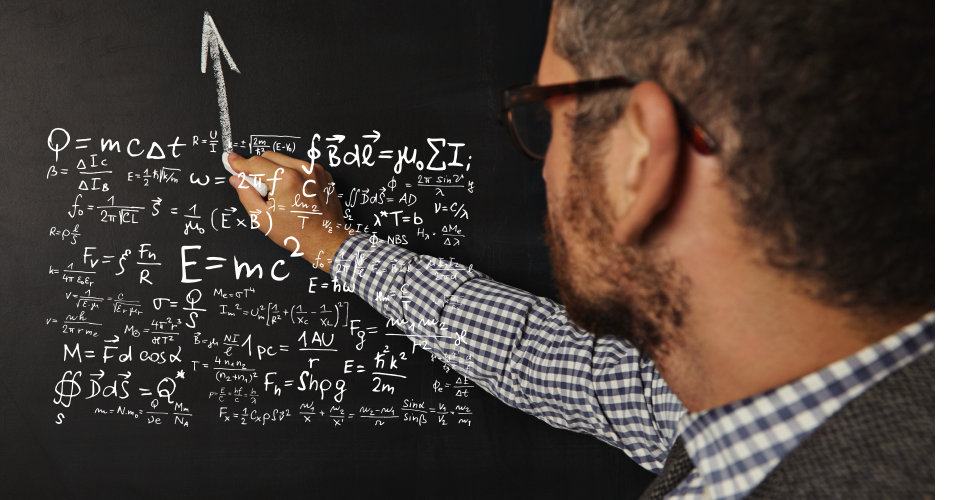• Save

# SSC CGL Maths Questions Bank 2021

Vikram Singh2 months ago 482 Views
NEWMathematics is an important and scoring subject for SSC CGL exam. Sometimes students take a lot of time to solve and understand maths questions in the exam, which can only be made successful with maximum practice of CGL maths questions.

So, to avoid wastage of time, you should move towards SSC CGL Maths Questions Bank 2021 given here. To crack the CGL exam, students must practice these maths questions and answers regularly, which is useful for the upcoming SSC CGL exam 2021.

Go to SSC CGL Test Series here for more practice.

## SSC CGL Maths Practice Questions

Q :

A and B can together complete a task 40 days. They worked together for 30 days and then left. A finished the remaining task in next 22 days. In how many days A alone can finish the task?

(A) 88 days

(B) 48 days

(C) 30 days

(D) 40 days

Q :

In bag there are coins of 25 paisa, 50 paisa and 1 rupee in the ratio of 3 : 4 : 5. If there are in all Rs.31, then how many 1 rupee coins are there in the bag?

(A) 12

(B) 16

(C) 24

(D) 20

Q :

Cost of two articles were in the ratio of 16: 23. The cost of first article increases by 10% and that of second by Rs.477. Now the costs of two articles are in the ratio of 11: 20. The price of the second article (in Rs.) in the beginning was-

(A) 1912

(B) 1251

(C) 1521

(D) 1219

Q :

A reduction of 20% in the price of sugar enables a housewife to purchase 6 kg. more for Rs. 240. What is the original price per kg. of sugar?

(A) Rs. 25 per kg

(B) Rs. 18 per kg

(C) Rs.15 per kg

(D) Rs. 10 per kg

Q :

Anoop travels first 1/3 of the total distance at the speed of 10 km/hr. and the next 1/3 distance at the speed of 20 km/hr. and the last 1/3 distance at the speed of 60 km/hr. The average speed of Anoop is-

(A) 18

(B) 21

(C) 14

(D) 16

Q :

A train 400 m long passes a pole in 20 seconds. What is the speed of the train?

(A) 70 km/hr

(B) 68 km/hr

(C) 72 km/hr

(D) 64 km/hr

Q :

A train 300 m. long is running with a speed of 54 km/hr. In what time will it cross a telephone pole?

(A) 16 sec

(B) 20 sec

(C) 18 sec

(D) 22 sec

Q :

A train passes a 100 meters long platform in 28 seconds and a man standing on the platform in 20 seconds. The speed of the train is:

(A) 50 km./hr

(B) 45 km./hr

(C) 40 km./hr

(D) 60 km./hr

Q :

A sum of money was invested at a certain rate of simple interest for 2 years. Had it been invested at 1% higher rate, it would have fetched Rs.192 more interest. The sum of money is-

(A) Rs.9000

(B) Rs.9600

(C) Rs.7200

(D) Rs.8400

Q :

An amount doubles itself in 5 years simple interest. What is the rate of interest per year?

(A) 20%

(B) 35 %

(C) 25 %

(D) Cannot be determined